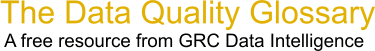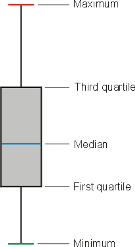Choose Index below for a list of all words and phrases defined in this glossary.

# Box Plot

index | Index

## Box Plot - definition(s)

box plot - A box plot is a graphical rendition of statistical data based on the minimum, first quartile, median, third quartile, and maximum. The term "box plot" comes from the fact that the graph looks like a rectangle with lines extending from the top and bottom. Because of the extending lines, this type of graph is sometimes called a box-and-whisker plot.

In a typical box plot, the top of the rectangle indicates the third quartile, a horizontal line near the middle of the rectangle indicates the median, and the bottom of the rectangle indicates the first quartile. A vertical line extends from the top of the rectangle to indicate the maximum value, and another vertical line extends from the bottom of the rectangle to indicate the minimum value. The illustration shows a generic example of a box plot with the maximum, third quartile, median, first quartile, and minimum values labeled. The relative vertical spacing between the labels reflects the values of the variable in proportion.A box plot can be placed on a coordinate plane resembling the Cartesian system, so that the five values, arranged vertically one above the other, run parallel to the dependent-variable or y axis. In some situations, two or more box plots can be placed side-by-side on a Cartesian coordinate plane to show how a phenomenon or scenario evolves with time, which is plotted along the independent-variable or x axis. Once in a while, a single box plot is tilted on its side, so the values run from left-to-right (minimum to maximum) instead of bottom-to-top.

[Category=Data Management ]

Source: WhatIs.com, 08 July 2013 11:21:00, http://whatis.techtarget.com/glossary/Data-and-Data-ManagementData Quality Glossary.  A free resource from GRC Data Intelligence. For comments, questions or feedback: dqglossary@grcdi.nl